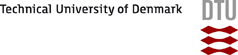DTU Risø Campus# Primary Yields

A G-value is defined as the number of molecules available for homogeneous chemical reactions per 100 eV absorbed radiation energy.

The G-values varies with LET, temperature, dose rate and solute concentration. The survey article (A.J. Elliot, Rate Constants and G-Values for the Simulation of the Radiolysis of Light Water over the Range 0-300ºC, AECL-11073, COG-94-167) is recommended as an introduction to the subject.

A set of G-values must in itself be in mass balance and charge balance.

As an example the G-values for gamma radiation of light water, pH 7 and room temperature are:

 G(OH) = 2.67 G(e-) = 2.66 G(H) = 0.55 G(H2) = 0.45 G(H2O2) = 0.72 G(H+) = 2.76 G(OH-) = 0.1 G(H2O) = -6.87

Note that e- contains one water molecule.

## Authors

 Peter Kirkegaard Applied Mathematician, Ph.D.DTU - Risø Campus, Roskilde, Denmark Erling Bjergbakke Bjergbakke Chemical ConsultingRoskilde, Denmark Jens V. Olsen System Programming AnalystDTU - Risø Campus, Roskilde, Denmark

Webmaster 5 Jul 2016